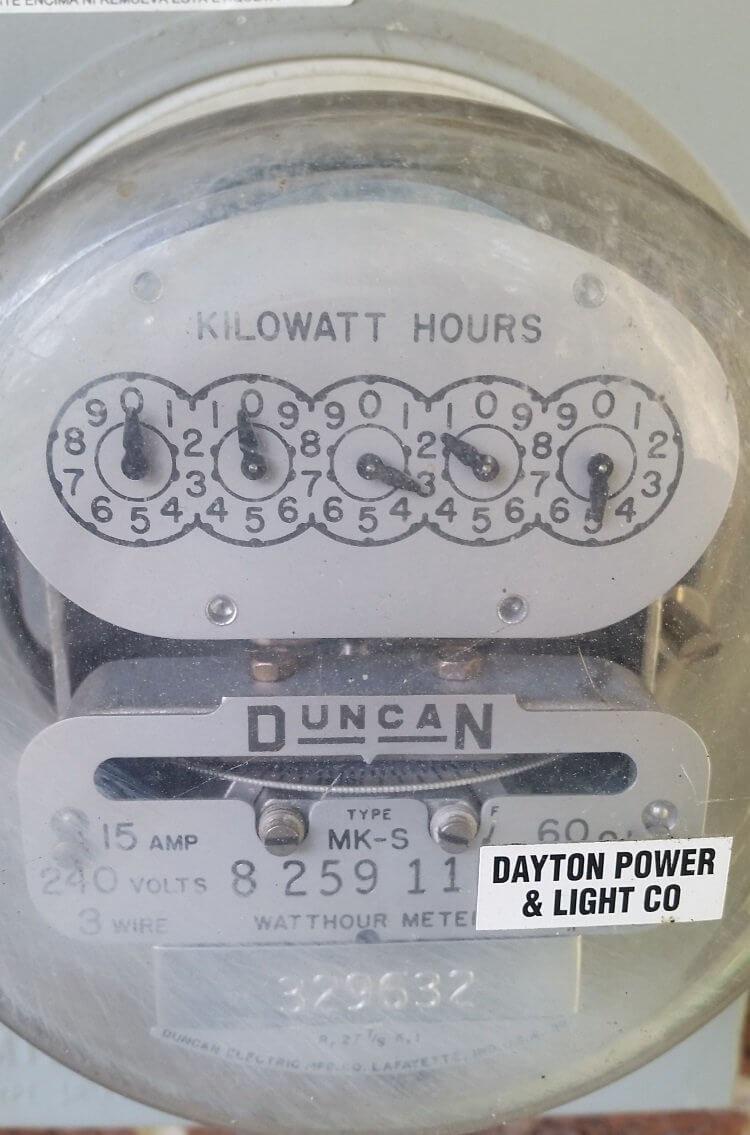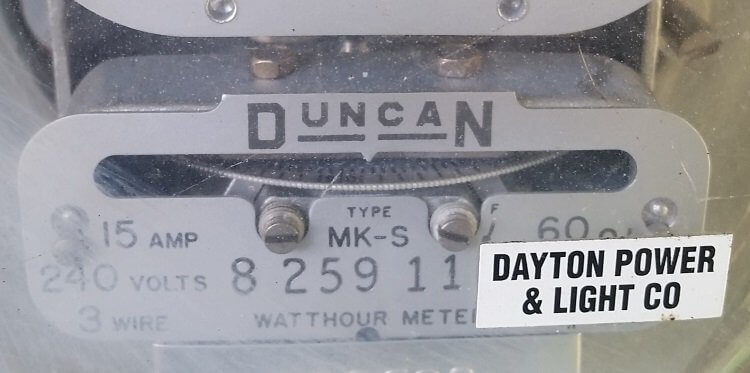I was curious as to what my instantaneous electrical usage was. Unfortunately, most resources I found online, such as WikiHow and various blog posts only told me how to reach the current usage on an analog electric meter.Think of current usage like the odometer on your car. If you want to know how long you traveled in a day, you take the odometer reading at the end of the day and subtract it from the reading at beginning of the day. But the odometer isn’t very good at letting you know how fast you’re going - for that you need a speedometer. So is there an equivalent to a speedometer on an electric meter?

Sadly, no, at least not on my analog electric meter. There is, however, a little disc that spins perpendicular to the rest of the dials. This disc makes one complete rotation for every watt-hour you use. This means it spins 1,000 times for every kilowatt-hour you use.

### The Confusing Unit Kilowatt-Hour

To understand why a kilowatt-hour is confusing, let’s look at one way to calculate it. If you use a device that uses one kilowatt for one hour, you use one kilowatt-hour. If another device uses 10 kilowatts for two hours, it uses 20 kilowatt-hours. That is, you multiply the number of kilowatts used by a device by the number of hours it is running.

A kilowatt is 1,000 joules per second. By multiplying a kilowatt by a time (in this case, hours), you’re canceling out the time in the unit itself (in this case, seconds). Intuitively (at least to me) it would make more sense to just use joules (or kilojoules, megajoules, etc). Instead, we use a unit that is equal to 3,600,000 joules, or 3.6 megajoules. That is, kilowatt-hours and joules are measuring the same thing (energy).

There are historical and practicality reasons for this (the joule itself is a unit that over time - actually seconds squared). In fact, there even is the unit of watt-second, which is exactly one joule.

### Back to Figuring Out Instantaneous Usage

The simple trick to figuring out instantaneous usage is to measure how fast the little disc is spinning. The easiest way to do this is to time it. Now, this isn’t exactly instantaneous, but it’s pretty close.

The little disc should have markings on it. These markings are little ticks, not unlike you find on a ruler or tape measure. Mine has a large black mark at the zero point, and numbers for every tenth location.

To time it, you just need a stopwatch. This can be an actual watch, or you can use a cell phone app. Start the timer when you see the zero point cross a little pointer above it. Stop the timer when it crosses the pointer again.For me, I got 13.3 seconds. That means I’m currently using one watt-hour every 13.3 seconds. So, uh, how do I convert this to something useful?

For starters, using one watt-hour every 13.3 seconds means you’re using a little more than 0.075 watt-hours every second (just divide 1 by 13.3). If you want to know how many watt-hours you use in a minute, divide 60 by 13.3 (which happens to be a little more than 4.5 watt-hours). If you want to know how many watt-hours you use in a month, divide the number of seconds in a month by 13.3.

Except that the length of a month is a bit ambiguous - it can be 28, 29, 30, or 31 days. For the purpose of this exercise let’s say a month is 30 days. That means a month is 30 days × 24 hours × 60 minutes × 60 seconds = 2,592,000 seconds. Therefore, at this rate, I’ll use 194,887.2 watt-hours in a month.

How many kilowatt-hours is that? Just divide by 1,000. Therefore I am energy at a rate of 194.9 kilowatt-hours a month.

Alternatively, you could just divide by 2,592 to begin with.

Finally, I took a couple more measurements just for fun. When my wife was cooking dinner the electric meter’s disc rotated in 6 seconds. Then I turned the air conditioner on and the disc spun in 2 seconds - which was really fast.

### Calculating Current Kilowatt Usage

To figure out the current kilowatt usage, simply divide 3.6 by the number of seconds it took for the electric meter’s disc to spin. Essentially what your doing is dividing the number of seconds in an hour (3,600) by the seconds the disc took to spin, and then dividing that number by 1,000.